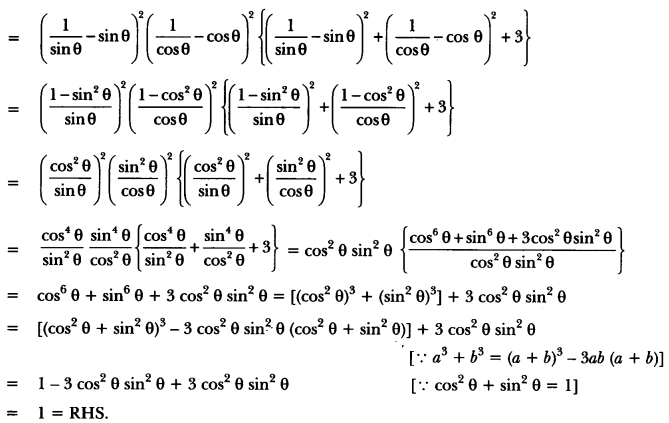# Introduction to Trigonometry Class 10 Extra Questions Maths Chapter 8 with Solutions Answers

Here we are providing Introduction to Trigonometry Class 10 Extra Questions Maths Chapter 8 with Answers Solutions, Extra Questions for Class 10 Maths was designed by subject expert teachers.

## Extra Questions for Class 10 Maths Introduction to Trigonometry with Answers Solutions

Extra Questions for Class 10 Maths Chapter 8 Introduction to Trigonometry with Solutions Answers

### Introduction to Trigonometry Class 10 Extra Questions Short Answer Type 1

Question 1.
Find maximum value of $$\frac{1}{\sec \theta}$$, 0°≤ θ ≤ 90°.
Solution:
$$\frac{1}{\sec \theta}$$, (0° ≤ θ ≤ 90°) (Given)
∵ sec θ is in the denominator
∴ The min. value of sec θ will return max. value for $$\frac{1}{\sec \theta}$$.
But the min. value of sec θ is sec 0° = 1.
Hence, the max. value of $$\frac{1}{\sec 0^{\circ}}$$ = $$\frac{1}{1}$$ = 1

Question 2.
Given that sin θ = $$\frac{a}{b}$$, find the value of tan θ.
Solution:
sin θ = $$\frac{a}{b}$$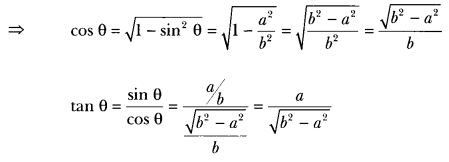Question 3.
If sin θ = cos θ, then find the value of 2 tan θ + cos2 θ.
Solution:
sin θ = cos θ (Given)
It means value of θ = 45°
Now, 2 tan θ + cos2 θ = 2 tan 45° + cos2 45°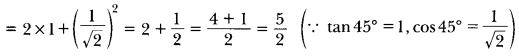Question 4.
If sin (x – 20)° = cos (3x – 10)°, then find the value of x.
Solution:
sin (x – 20)° = cos (3x – 10)°
⇒ cos [90° – (x – 20)°] = cos (3x – 10)°
By comparing the coefficient
90° – x° + 20° = 3x° – 10° = 110° + 10° = 3x° + x°
120° = 4x°
⇒ $$\frac{120^{\circ}}{4}$$ = 30°

Question 5.
If sin2 A = $$\frac{1}{2}$$tan2 45°, where A is an acute angle, then find the value of A.
Solution:
sin2A = $$\frac{1}{2}$$tan2 45°
⇒ sin2A = $$\frac{1}{2}$$ (1)2 [∵ tan 45° = 1]
= sin2 A = $$\frac{1}{2}$$
⇒ sin A = $$\frac{1}{\sqrt{2}}$$
Hence, ∠A = 45°

Question 6.
If x = a cos θ, y = b sin θ, then find the value of b2x2 + a2y2 – a2b2.
Solution:
Given x = acos θ, y = b sin θ
b2x2 + a2y2 – a2b2 = b2(acos θ)2 + a2(b sin θ)2 – a2b2
= a2b2 cos2θ + a2b2 sin2 θ – a2b2 = a2b2 (sin2 θ + cos2 θ) – a2b2
= a2b2 – a2b2 = θ (∵ sin2 θ + cos2 θ = 1)

Question 7.
If tan A = cot B, prove that A + B = 90°.
Solution: We have
tan A = cot B
⇒ tan A = tan (90° – B)
A = 90° – B
[∵ Both A and B are acute angles]
⇒ A + B = 90°

Question 8.
If sec A = 2x and tan A = $$\frac{2}{x}$$, find the value of 2$$\left(x^{2}-\frac{1}{x^{2}}\right)$$ .
Solution: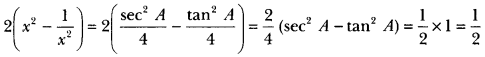Question 9.
In a ∆ABC, if ∠C = 90°, prove that sin2 A + sin2 B = 1.
Solution:
Since ∠C = 90°
∴ ∠A + ∠B = 180° – ∠C = 90°
Now, sin2 A + sin2 B = sin2 A + sin2 (90° – A) = sin2 A + cos2 A = 1

Question 10.
If sec 4A = cosec (A – 20°) where 4 A is an acute angle, find the value of A.
Solution:
We have
sec 4 A = cosec (A – 20°)
⇒ cosec (90° – 4 A) = cosec (A – 20°)
∴ 90° – 4 A = A – 20°
⇒ 90° + 20° = A + 4 A
⇒ 110° = 5 A
∴ A = $$\frac{110}{5}$$ = 22°

### Introduction to Trigonometry Class 10 Extra Questions Short Answer Type 2

Question 1.
If sin A = $$\frac{3}{4}$$, calculate cos A and tan A.
Solution:
Let us first draw a right ∆ABC in which ∠C = 90°.
Now, we know that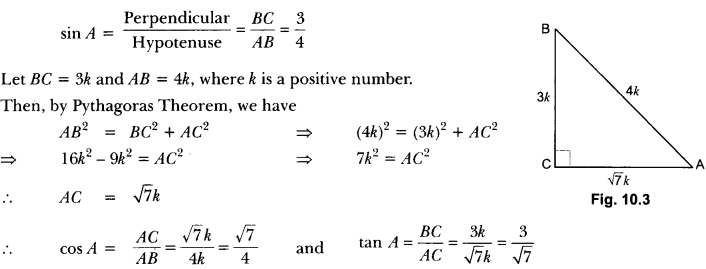Question 2.
Given 15 cot A = 8, find sin A and sec A.
Solution:
Let us first draw a right ∆ABC in which ∠B = 90°.
Now, we have, 15 cot A = 8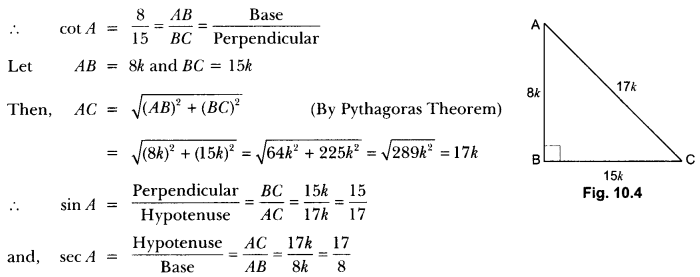Question 3.
In Fig. 10.5, find tan P – cot R.
Solution: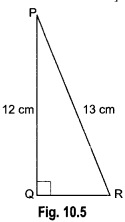Using Pythagoras Theorem, we have
PR2 = PO2 + QR2
⇒ (13)2 = (12)2 + QR2
⇒ 169 = 144 + QR2
⇒ QR2 = 169 – 144 = 25
⇒ QR = 5 cm
Now, tan P = $$\frac{QR}{PQ}$$ = $$\frac{5}{12}$$ and cot R = $$\frac{QR}{PQ}$$ = $$\frac{5}{12}$$
tan P – cot R = $$\frac{5}{12}$$ – $$\frac{5}{12}$$ = 0

Question 4.
If sin θ + cos θ = √3 , then prove that tan θ + cot θ = 1.
Solution:
sin θ + cos θ = √3
⇒ (sin θ + cos θ)2 = 3
⇒ sin2 θ + cos2 θ + 2 sin θ cos θ = 3
⇒ 2 sin cos θ = 2 (∵ sin2 θ + cos2 θ = 1)
⇒ sin θ. cos θ = 1 = sin2 θ + cos2 θ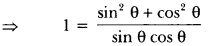⇒ 1 = tan θ + cot θ = 1
Therefore tan θ + cot θ = 1

Question 5.
Prove that $$\frac { 1-sinθ }{ 1+sinθ }$$ = (sec θ – tan θ)2
Solution: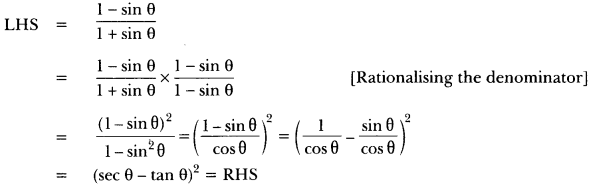Without using tables, evaluate the following (6 to 10).

Question 6.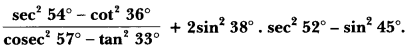Solution: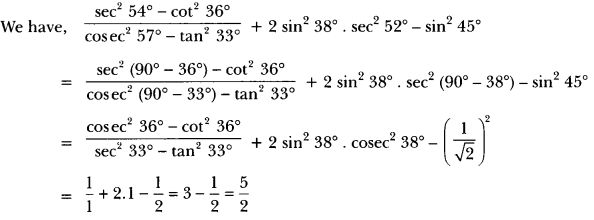Question 7.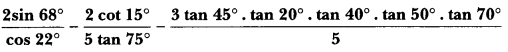Solution: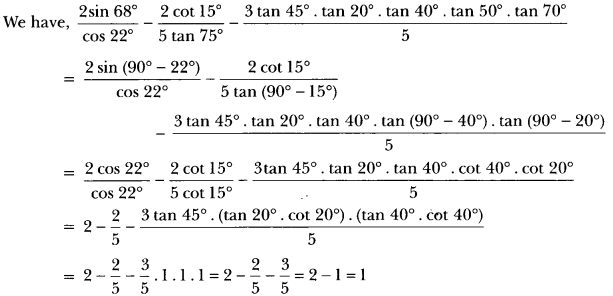Question 8.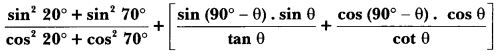Solution: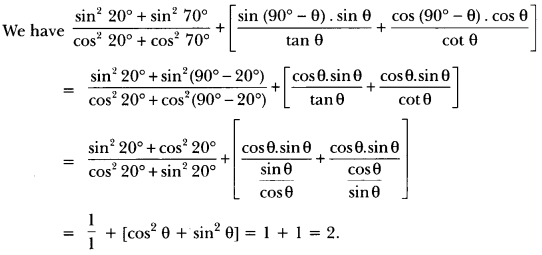Question 9.
Evaluate: sin 25° cos 65° + cos 25° sin 65°.
Solution:
sin 25°. cos 65° + cos 25° . sin 65°
= sin (90° – 65°). cos 65° + cos (90° – 65°). sin 65°
= cos 65° . cos 65° + sin 65°. sin 65°
= cos2 65° + sin2 65° = 1.

Question 10.
Without using tables, evaluate the following:
3 cos 68°. cosec 22° – $$\frac{1}{2}$$ tan 43°. tan 47°. tan 12°. tan 60°. tan 78°
Solution:
We have,
3 cos 68°. cosec 22° – $$\frac{1}{2}$$ tan 43°. tan 47°. tan 12°. tan 60°. tan 78°.
= 3 cos (90° – 22°). cosec 22° – $$\frac{1}{2}$$ . {tan 43° . tan (90° – 43°)}. {tan 12°. tan (90° – 12°). tan 60°}
= 3 sin 22°. cosec 22° – $$\frac{1}{2}$$(tan 43° . cot 43°). (tan 12°. cot 12°). tan 60°
= 3 × 1 – × 1 × 1 × √3 = 3 – $$\frac{\sqrt{3}}{2}$$ = $$\frac{6-\sqrt{3}}{2}$$.

Question 11.
If sin 30 = cos (θ – 6°) where 30 and (θ – 6°) are both acute angles, find the value of θ.
Solution:
According to question:
sin 3θ = cos (θ – 6°)
cos (90° – 30) = cos (θ – 6°) [∵ cos (90° – θ ) = sin θ]
90° – 3θ = θ – 6° [comparing the angles)
= 4θ = 90° + 6° = 96°
θ = $$\frac{96}{4}$$ = 24°
Hence, θ = 24°

Question 12.
If sec θ = x + $$\frac{1}{4x}$$, prove that sec θ + tan θ = 2x or $$\frac{1}{2x}$$.
Solution:
Let sec θ + tan θ = λ …..(i)
We know that, sec2 θ – tan2 θ = 1
(sec θ + tan θ) (sec θ – tan θ) = 1
⇒ λ(sec θ – tan θ) = 1
sec θ – tan θ = $$\frac{1}{\lambda}$$ …..(ii)
Adding equations (i) and (ii), we get

Question 13.
Find an acute angle θ, when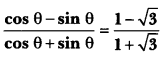Solution: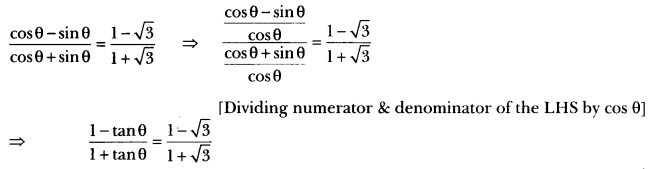On comparing we get
⇒ tan θ = √3
⇒ tan θ = tan 60°
= θ = 60°

Question 14.
The altitude AD of a MABC, in which ∠A is an obtuse angle has length 10 cm. If BD = 10 cm and CD = 10√3 cm, determine ∠A.
Solution: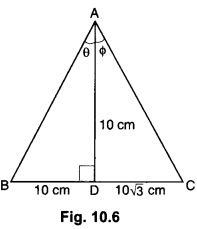∆ABD is a right triangle right angled at D, such that AD = 10 cm and BD = 10 cm.
∴ tan θ = $$\frac{B D}{A D}$$
⇒ tan θ = $$\frac{10}{10}$$ = 1
⇒ tan θ = tan 45°
⇒ θ = ∠BAD = 45° … (i)
∆ACD is a right triangle right angled at D such that AD = 10 cm and DC = 10√3 cm.
∴ tan Φ = $$\frac{CD}{AD}$$
⇒ tan Φ = $$\frac{10 \sqrt{3}}{10}$$ = √3
⇒ tan Φ = tan 60°
⇒ Φ = ∠CAD = 60°
From (i) & (ii), we have

Question 15.
If cosec θ = $$\frac{13}{12}$$, evaluate $$\frac{2 \sin \theta-3 \cos \theta}{4 \sin \theta-9 \cos \theta}$$
Solution: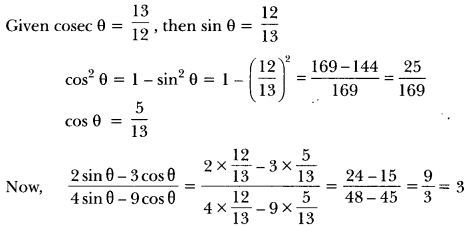Question 16.
Prove that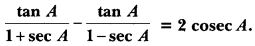Solution: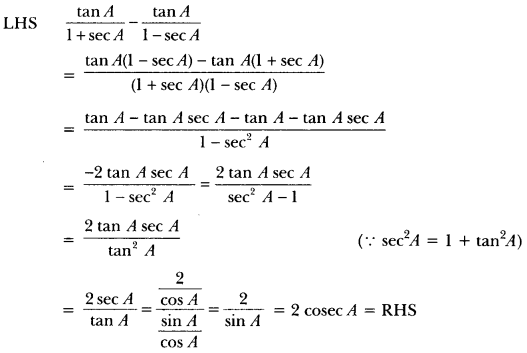Question 17.
Prove that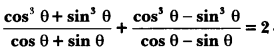Solution:
We have,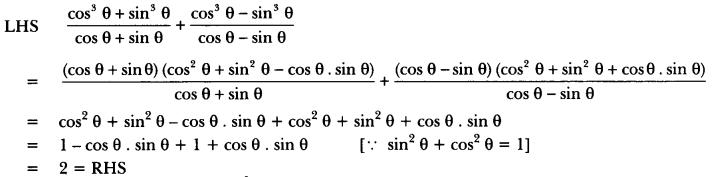Question 18.
Prove that: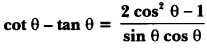Solution: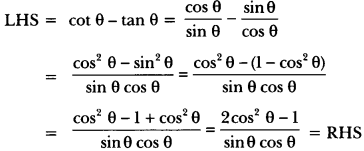Question 19.
Prove that: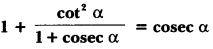Solution: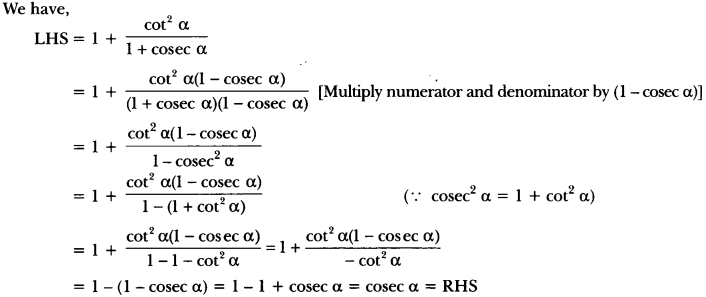Question 20.
Evaluate the following: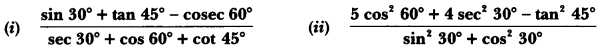Solution: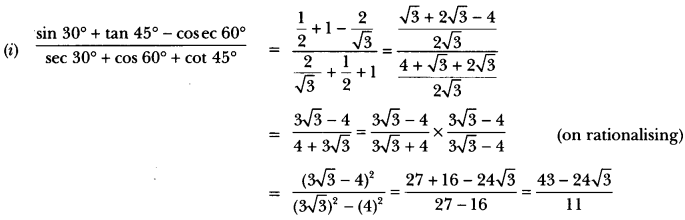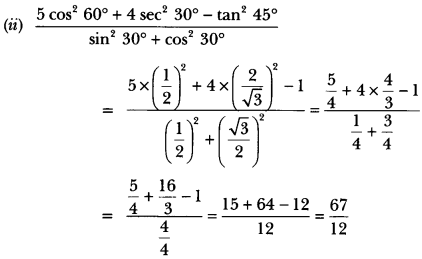Question 21.
If tan (A +B) = √3 and tan (A – B) = $$\frac{1}{\sqrt{3}}$$; 0° < A + B ≤ 90°; A > B, find A and B.
Solution:
We have, tan (A + B) = √3
⇒ tan (A + B) = tan 60°
∴ A + B = 60° …(i)
Again, tan (A – B) = $$\frac{1}{\sqrt{3}}$$
∴ A – B = 30° … (ii)
Adding (i) and (ii), we have
2A = 90°
⇒ A = 45°
Putting the value of A in (i), we have
45° + B = 60°
∴ B = 60° – 45o = 15°
Hence, A = 45° and B = 15°

Question 22.
If A, B and C are interior angles of a ∆ABC, then show that sin $$\left(\frac{B+C}{2}\right)$$ = cos$$\frac{A}{2}$$.
Solution:
Since A, B and C are the interior angles of a ∆ABC,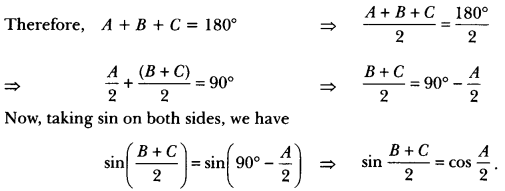Question 23.
Prove that: (cosec θ – cot θ)2 = $$\frac {1-\cos\theta}{1+\cos\theta}$$
Solution:
LHS = (cosec θ – cot θ)2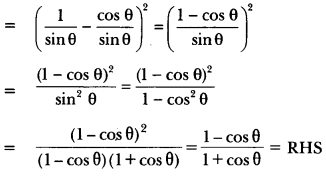Question 24.
Prove that: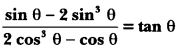Solution: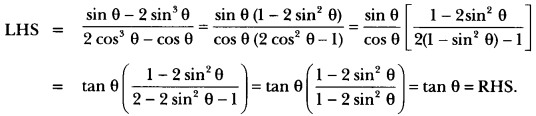Question 25.
Prove that: (sin A + cosec A)2 + (cos A + sec A)2 = 7 + tan2 A + cot2A.
Solution:
LHS = (sin A + cosec A)2 + (cos A + sec A)2
= sin2 A + coses2 A + 2sin A . cosec A + cos2 A + sec2 A + 2 cos A . sec A
= (sin2 A + coses2 A + 2) + (cos2 A + sec2 A + 2) [sin A. cosec A = 1]
= (sin2 A + cos2 A) + (coses2 A + sec2 A) + 4 [cos A. sec A = 1]
= 1 + 1 + cot2A + 1 + tan2 A + 4
= 7 + tan2 A + cot2 A = RHS [∵ 1 + cot2A = coses2 A and 1 + tan2 A = sec2 A]

Question 26.
Prove that: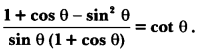Solution: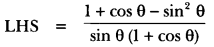To obtain cot θ in RHS, we have to convert the numerator of LHS in cosine function and denominator in sine function.
Therefore converting sin2 θ = 1 – cos2 θ, we get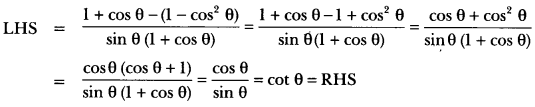Question 27.
Prove that: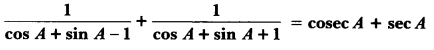Solution: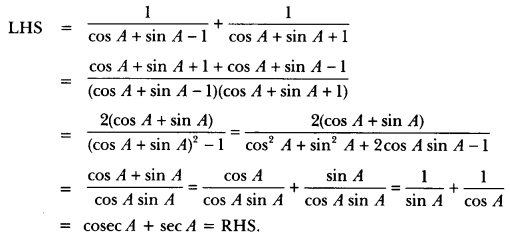Question 28.
Prove that: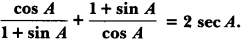Solution: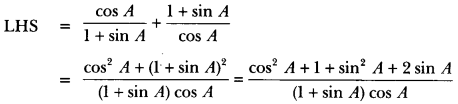Question 29.
Without using trigonometric tables, prove that: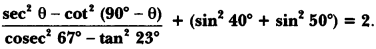Solution: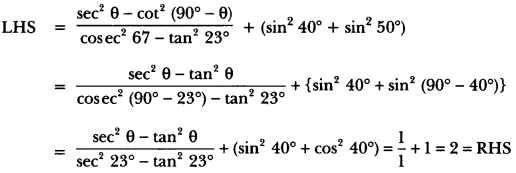Question 30.
Evaluate: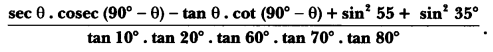Solution: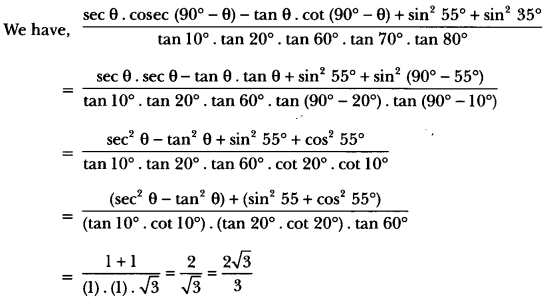### Introduction to Trigonometry Class 10 Extra Questions Long Answer Type

Question 1.
In ∆PQR, right-angled at Q, PR + QR = 25 cm and PQ = 5 cm. Determine the values of sin P, cos P and tan P.
Solution: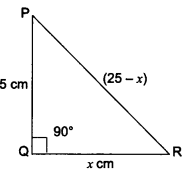We have a right-angled ∆PQR in which ∠Q = 90°.
Let QR = x cm
Therefore, PR = (25 – x) cm
By Pythagoras Theorem, we have
PR2 = PQ2 + QR2
(25 – x)2 = 52 + x2
= (25 – x)2 – x2 = 25
(25 – x – x) (25 – x + x) = 25
(25 – 2x) 25 = 25
25 – 2x = 1
25 – 1 = 2x
= 24 = 2x
∴ x = 12 cm
Hence, QR = 12 cm
PR = (25 – x) cm = 25 – 12 = 13 cm
PQ = 5 cm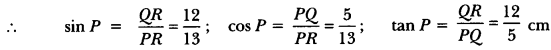Question 2.
In triangle ABC right-angled at B, if tan A = $$\frac{1}{\sqrt{3}}$$ find the value of:
(i) sin A cos C + cos A sin C (ii) cos A cos C – sin A sin C.
Solution: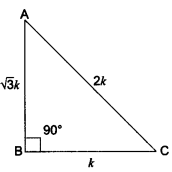We have a right-angled ∆ABC in which ∠B = 90°.
and, tan A = $$\frac{1}{\sqrt{3}}$$
Now, tan A = $$\frac{1}{\sqrt{3}}$$ = $$\frac{BC}{AB}$$
Let BC = k and AB = √3k
∴ By Pythagoras Theorem, we have
⇒ AC2 = AB2 + BC2
⇒ AC2 = (√3k)2 + (k)2 = 3k2 + k2
⇒ AC2 = 4k2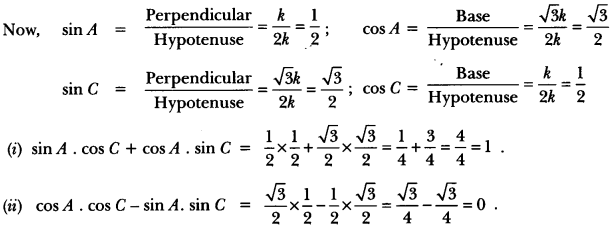Question 3.
If cot θ = $$\frac{7}{8}$$, evaluate:
(i)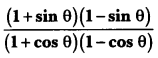(ii) cot2 θ
Solution:
Let us draw a right triangle ABC in which ∠B = 90° and ∠C = θ.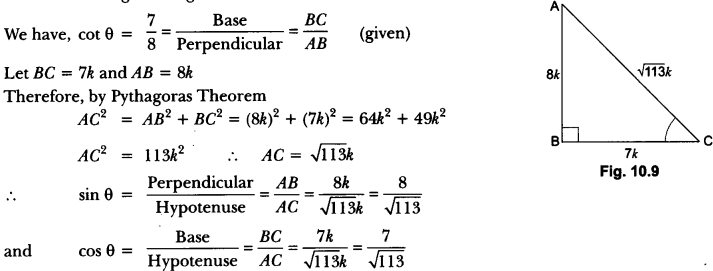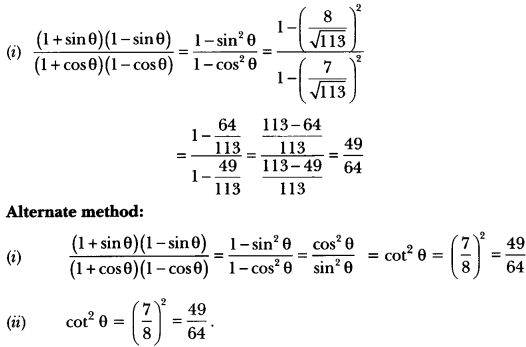Question 4.
If 3 cot A = 4, check whether $$\frac{1-\tan ^{2} A}{1+\tan ^{2} A}$$ = cos2 A – sin2 A or not.
Solution: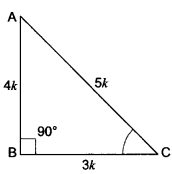Let us consider a right triangle ABC in which ∠B = 90°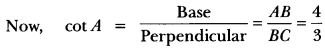Let AB = 4k and BC = 3k
∴ By Pythagoras Theorem
AC2 = AB2 + BC2
AC = (4k)2 + (3k)2 = 16k2 + 9k2
AC2 = 25k2
∴ AC = 5k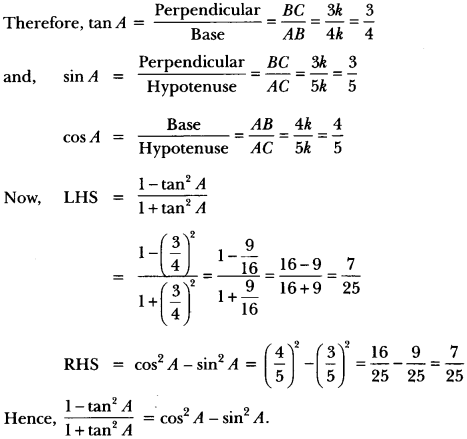Question 5.
Write all the other trigonometric ratios of ∠A in terms of sec A.
Solution: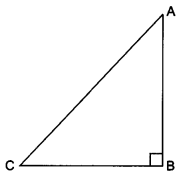Let us consider a right-angled ∆ABC in which ∠B = 90°.
For ∠A we have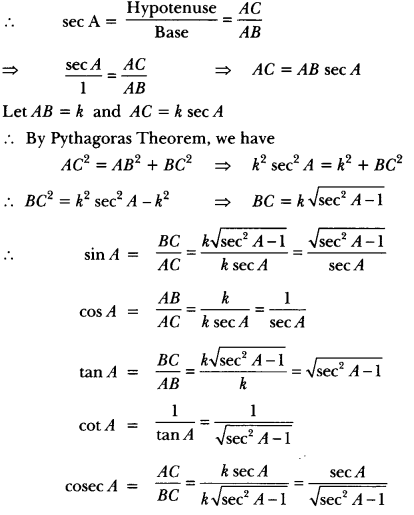Question 6.
Prove that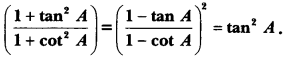Solution: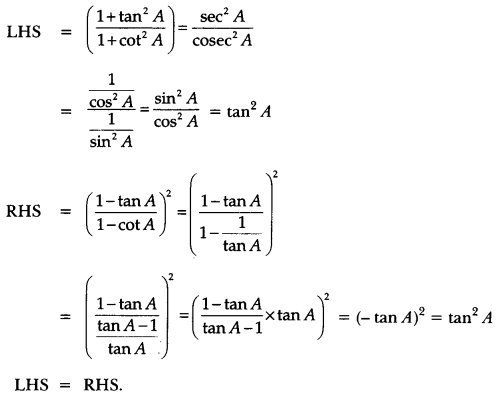Question 7.
Prove that: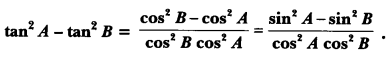Solution: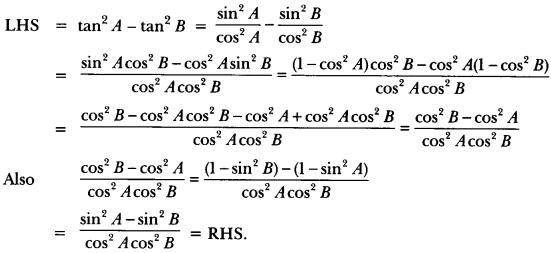Question 8.
Prove that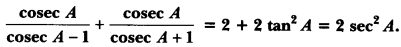Solution: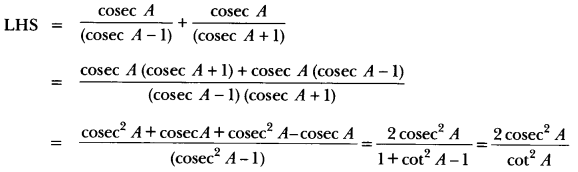= 2 cosec2 A tan2 A = 2(1 + cot2 A). tan2 A
= 2 tan2 A + 2 tan2 A. cot2 A (∵ tan A cot A = 1)
= 2 + 2 tan2 A = 2(1 + tan2 A) = 2 sec2 A = RHS.

Question 9.
Prove that: (sin θ + sec θ)2 + (cos θ + cosec θ)2 = (1 + sec θ cosec θ)2.
Solution:
LHS = (sin θ + sec θ)2 + (cos θ + cosec θ)2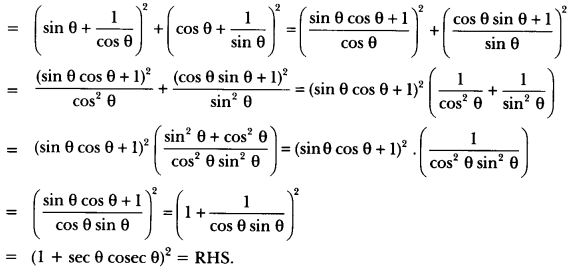= (1 + sec θ cosec θ)2 = RHS.

Question 10.
Prove that: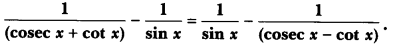Solution:
In order to show that,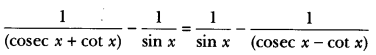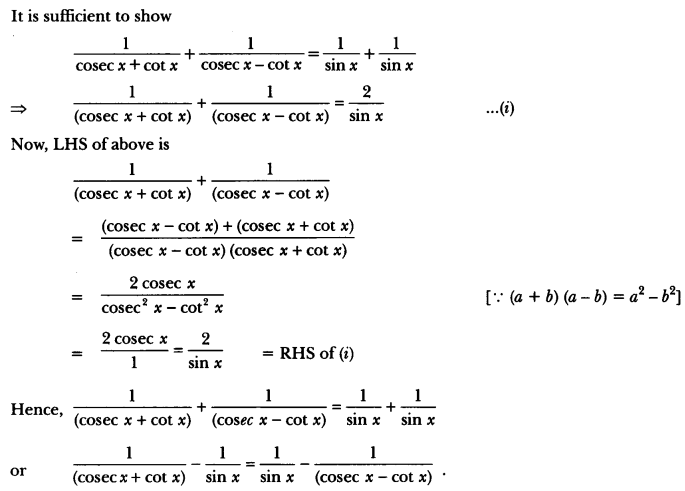Question 11.
Prove that: $$\frac { cosecθ\quad +\quad cotθ }{ cosecθ\quad -\quad cotθ }$$ = (cosec θ + cot θ) = 1 + 2 cot2 θ + 2 cosec θ cot θ.
Solution: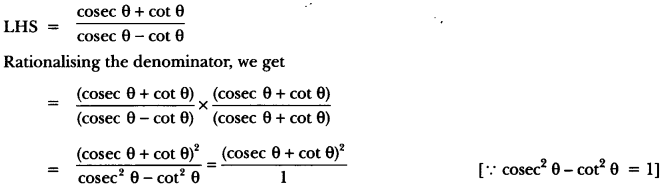= cosec2 θ + cot2 θ + 2 cosec θ. cot θ
= (1 + cot2 θ) + cot2 θ + 2 cosec θ. cot θ
= 1 + 2 cot2 θ + 2 cosec . cot θ = RHS.

Question 12.
Prove that: 2 sec θ – sec θ – 2 cosec θ + cosec θ = cot – tan θ.
Solution:
LHS = 2 sec θ – sec θ – 2 cosec2 θ + cosec θ
= 2 (sec2 θ) – (sec2 θ)2 – 2 (cosec2 θ) + (cosec θ)2
= 2 (1 + tan2 θ) – (1 + tan4 θ)2 – 2(1 + cot2 θ) + (1 + cot2 θ)4
= 2 + 2 tan2 θ – (1 + 2 tan2 θ + tan2 θ) – 2 – 2 cot2 θ + (1 + 2 cot2 θ + cot θ) =
= 2 + 2 tan2 θ – 1 – 2 tan2 θ – tan4 θ – 2 – 2 cot2 θ + 1 + 2 cot4 θ + cot4 θ
= cot4 θ – tan4 θ = RHS

Question 13.
Prove that: (cosec A – sin A) (sec A – cos A) = $$\frac{1}{\tan A+\cot A}$$.
Solution:
LHS = (cosec A – sin A) (sec A – cos A)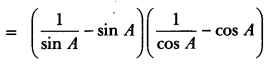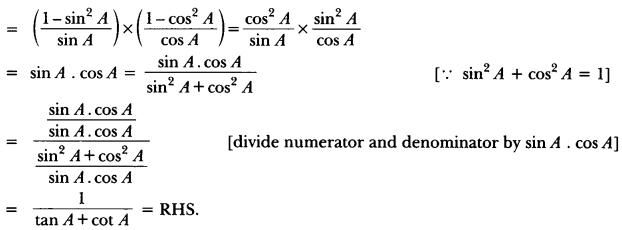### Introduction to Trigonometry Class 10 Extra Questions HOTS

Question 1.
Prove that:
$$\frac{\tan \theta}{1-\cot \theta}$$ + $$\frac{\cot \theta}{1-\tan \theta}$$ = 1 + sec θ cosec θ = 1 + tan θ + cot θ.
Solution: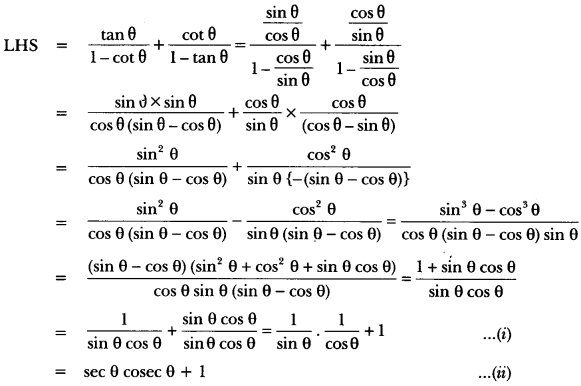For second part
Now from (i), we have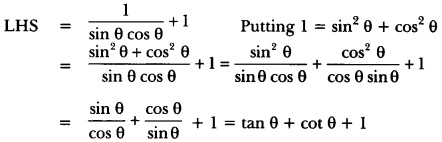Question 2.
If tan A = n tan B and sin A = m sin B, prove that cos2 A = $$\frac{m^{2}-1}{n^{2}-1}$$
Solution:
We have to find cos2 A in terms of m and n. This means that the angle B is to be eliminated from
the given relations.
Now, tan A = n tan B
⇒ tan B = $$\frac{1}{n}$$ tan A
⇒ cot B = $$\frac{n}{\tan A}$$
and
sin A = m sin B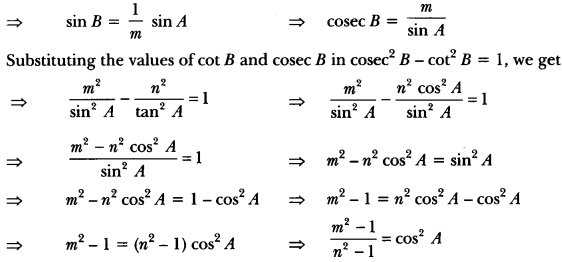Question 3.
Prove the following identity, where the angle involved is acute angle for which the expressions are defined.
$$\frac { cosA-sinA+1 }{ cos A + sin A-1 }$$ = cosec A + cot A, using the identity cosec2 A = 1 + cot2 A.
Solution: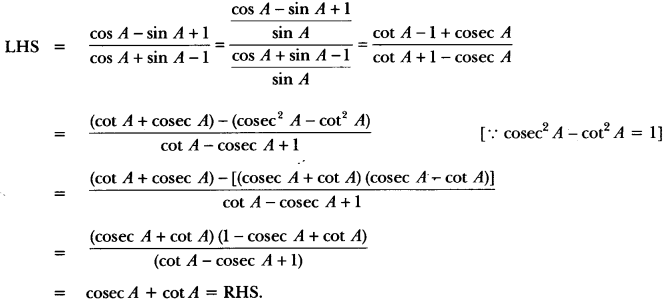= cosec A + cot A = RHS.

Question 4.
If x sin3 + y cos3 θ = sin θ cos θ and x sin θ = y cos θ, prove x2 + y2 = 1.
Solution:
We have, x sin3 + y cos3 θ = sin θ cos θ
⇒(x sin θ) sin2 θ + (y cos θ) cos2 θ sin θ cos θ
⇒ x sin θ (sin2 θ) + (x sin θ) cos2 = sin θ cos [∵ x sin θ = y cos θ]
⇒ x sin θ (sin2 θ + cos2 θ) = sin θ cos θ
⇒ x sin θ = sin θ cos
⇒ x = cos θ
Now, we have x sin θ = y cos θ
⇒ cos o sin θ = y cos θ [∵ x = cos θ]
⇒ y = sin θ
Hence, x2 + y2 = cos2 θ + sin2 θ = 1.

Question 5.
If tan θ + sin θ = m and tan θ – sin θ = n, show that (m2 – n2) = 4√mn.
Solution:
We have, given tan θ + sin θ = m, and tan θ – sin θ = n, then
LHS = (m2 – n2),= (tan θ + sin θ)2 – (tan θ – sin )2
= tan2 θ + sin2 θ + 2 tan θ sin θ – tan2 θ – sin2 θ + 2 tan θ sin θ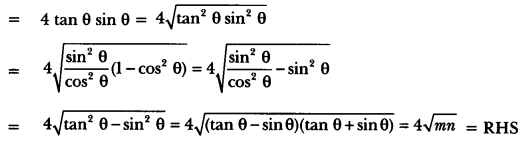Question 6.
If cosec θ – sin θ = l and sec θ – cos θ = m, prove that l2 m2 (l2 + m2 + 3) = 1.
Solution:
LHS = l2 m2 (l2 + m2 + 3).
= (cosec θ – sin θ)2 (sec θ – cos θ)2 {(cosec θ – sin θ)2 + (sec θ – cos θ)2 + 3}